The very definition of complexity is itself a complicated task. The root of the Latin word complex means “twisted or joined together” (from cum (together) + plecto (I put).  One can use the same words in Greek obtaining the word sum-plekw. Even if the meaning is the same, the english word symplexus indicates simply an ensemble of mathemical objects). Therefore just from the etimology similar to that of “complicated” (that is intertwingled) we must expect something difficult to characterize. Indeed no precise mathematical definition can be given for this word, but rather a fuzzy series of concepts that define an almost blurred idea.

Certainly, complexity refers to situations where many simple interacting parts produce a collective unexpected behaviour. This calls for another imprecise concept that is emergence.  Complex systems have emergence of new properties from the microscopical ones. Many examples are available.  Only restricting to physics one of the simplest is given by crystals.  There the atoms are rearranged in a regular structure. In this case, the electric field of nuclei forms a periodic potential for the electrons. The solutions of the Schroedinger equation  gives eventually rise to metals or insulators materials.

One important example of emergence is self-organization. The parts of a complex adaptive system, from grain of sands to living species can self-organize in a stable (in a statistical sense) state. The basic mechanism is that of feedback. Various parts communicate with their neighbours and arrange a common collective behaviour. Sometime, regardless the precise dynamics of the interactions, the evolution of the system is represented by some (in a statistical sense) stable state. This means that this steady state is an attractor in the phase space for the system dynamics and accounts for the robustness of complex systems with respect to external perturbation.

Since this intrinsic difficulty in definition of complexity, different scientists developed studies and investigation in this area along slightly different lines, and sometime the same word can indicate slightly different things.

Historically and philosophically the concept of complexity is rather recent. The beginning of scientific thought in ancient Greece was focused on the quest of the first principle (archè) from which everything has to descend. When Galileo and Newton founded the basis of Physics and the other Natural Sciences, they made use of the philosophical concept of determinism. This means that from a similar cause we must obtain a similar effect. Therefore any phenomenon, from the simple laboratory experiment to the evolution of the Universe must proceed without deviation from predetermined laws. Newton’s laws of dynamics are a set of simple recipes remarkably successful in predicting the motion of little objects on Earth as well as the motion of the Moon and the other planets. These laws are deterministic since once the initial conditions are given with infinite precision, then all the future evolution is completely determined. Given this scenario, complexity cannot exist. Given the initial condition nothing really unexpected can enter the game. Things are slightly different though and severe exception from determinism started to be evident at the end of 19 century.

I believe that all these approaches are the basis of the science of complexity, so it is worth to spend some time discussing all these exceptions from the dream of determinism.

To start with, knowing with infinite precision an initial state is only a limiting process, even worst one cannot increase the precision of a measurement ad libitum, since due to the postulate of Quantum Mechanism an intrinsic indetermination of the order of the Planck constant h is related to any measurement. The only hope is that a minimum error in the initial conditions reflects in a minimum error in the time evolution. This is not the case. Just at the beginning of 1900 the physicist Henri Poincaré became interested in the study of planetar motion and noticed that not always a little imprecision in the initial condition was related to a little imprecision in the determination of future behaviour. Rather, he was able to show (for a system of three or more interacting bodies) how a tiny error in the initial conditions could produce a remarkably large and fast growing difference in the future outcomes.

This means that starting from two sets of initial conditions (as similar as one can do) we can arrive in two completely different states for the same system (obeying the same laws). It does not matter the effort we put in the measurement of those conditions (Anyway at scale of h we have to stop) at the end the divergence of the final conditions is unavoidable. This peculiar sensitivity to initial conditions was called dynamical instability, or chaos. Since long-term mathematical predictions made for chaotic systems are as precise as a random prediction, the equations of motion can help only for a very short-term analysis.

This behaviour was considered at the beginning nothing more that a mathematical oddity. After some time, nevertheless, other phenomena were discovered to follow a similar behaviour. In the sixties the work of the meteorologist Edward Lorenz, showed how even a simple model of weather evolution possessed this chaotic behaviour. In his model an external source of heat (the sun) acts on the atmposphere generating air currents. The mathematical equations for the flow of the air gave unexpectedly very different behaviour for different sets of similar initial values. Even a microscopical difference can cause a completely different time evolution. This signature of chaos accounts for the difficulty of long-range weather forecast and was lately known with the popular name of “butterfly effects”. Indeed a butterfly flappings the wings in South America can produce after years a storm in central Asia. Thus the presence of chaotic systems in nature seems to place a limit on our ability to apply deterministic physical laws to predict motions with any degree of certainty.

The mathematical quantities measuring this divergence of the time evolution are called Lyapunov exponents. They quantify the rate of exponential divergence of nearby trajectories. The simplest approximation to the Lyapunov exponent is obtained by numerically computing the evolution of two trajectories that are initially nearly. So we get two (possibly high-dimensional) orbits (x(t), p(t)) and (x'(t), p'(t)). An approximate Lyapunov exponent is given by: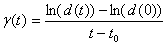where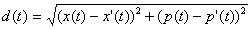is a possible distance definition between trajectories in phase space.  When t →∞ the larges Lyapunov exponent survives and g(t) →l , so that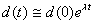The Lyapunov exponent is a measure of the exponent if the trajectories separate exponentially in time. It is zero for regular trajectories. The inverse of the Lyapunov exponent is the Lyapunov time. A technical detail is that once the two trajectories separate from one another they no longer test how nearby trajectories diverge. A better definition of the Lyapunov exponent is to compute one reference trajectory, and integrate the equations for linearized variations about this reference trajectory. This is the mathematic definition of the Lyapunov exponent, and it works well numerically.  Finally, another purely numerical technicality is that even the linearized variations can become too large to be represented in the computer. So it is convenient  to periodically store the size of g and rescale the variation to be again small (maintaining the direction). It is not good to rescale more than necessary since it introduces some noise in the determination of the value. It is also possible to use renormalization to solve the problem of saturation in the two trajectory method. All methods, if properly executed, give the same answers.

In the same years it became clear that determinism was also of no use to analyze large systems. One of the simplest possible interactions one can imagine is given by elastic scattering between particles. Since any macroscopic gas is essentially made of particles loosely interacting (mainly through scattering) it is possible to predict the behaviour of few cubic decimeter of gas by simultaneously solving the motion equations for the various particles. Apart the problem in the determination of the initial conditions described above, the incredibly huge number of particles (of the order of 10 raised to the power of 23), makes unrealistic any possibility to solve the problem in such a way. Blurring a little the concept of determinism one can now try to understand if at least statistical laws can be given in order to describe and predict the behaviour of these systems.  New quantities as Temperature and Pressure emerge. Thanks to the work of Ludwig Boltzmann and Joshua Gibbs we can have an insight of the connection between the microscopical interactions between the particles and these macroscopical quantities like pressure and temperature.  While in this case the emergence of new properties is particularly clear, one aspect of thermodynamics is even more connected to the idea of complexity.  Simple substances can coexist in different arrangements roughly speaking water can be in a liquid state or in a vapour state or in a solid state (actually more than one type of solid is present).  Experimentally by tuning external conditions one can drive the transformation of one phase in another with some kind of discontinuity either in the Gibbs energy or in the derivatives. Sometime instead as for the vapour liquid transition exists a point (critical point) after which transitions occur without discontinuity.  Nearby  the critical point the system start to behave in a very peculiar way. All the thermodynamical quantities start to diverge. The form of this divergence is that of a power-law, that is a law of the kind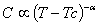This form is rather peculiar, since a power-law has no characteristical scale and it is often indicated as a scale-free relationship. This means that physically the system nearby the critical point behaves in a scale free fashion, where essentially the correlation extends on all the size of the system. Remarkably this behaviour is not reminiscent of the physical situation, applies to magnetic transitions, and phase transition for many substances. Such great universality must be related to fundamental physics, so we can try to capture this behaviour by means of a suitable model. The prototype of models is the Ising model where the system is supposed made of spin arranged on a regular structure (lattice).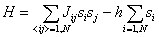The energy of the system is lowered for any spin aligning with the external field h, and spin interact with a specific interaction Jij . In the strong hypothesis of no eterogeneity at all the interactions are similar, Jij =-J for a ferromagnet and =J for an antiferromagnet.

The concept of scale-invariance allows using a powerful mathematical method called Renormalization Group. If the system appears the same at any scale then we can describe in various sizes expecting to find the same results.

In order to see how group works it is useful to define a paradigmatic model for phase transitions.

One of the keypoints of the onset of Statistical Mechanics is the fact that thermodynamical quantities are state functions, they depend on the present state of the system and not on the past history. Amongst the various potential, the energy has a key role since it defines the probability (Boltzmann weight) with which we can have a certain state. Unfortunately in other cases no Boltzmann weight is available, because the properties of the system depend on the past history of growth. A model of fractal growth is the Limited Diffusion Aggregation that has a very simple formulation. Starting from a seed other particles can attach to the cluster if in their random walk they happen to fall nearby the cluster.

Despite the simple formulation this recipe produces remarkably complicated objects whose probability of evolution depends interely on their past history.